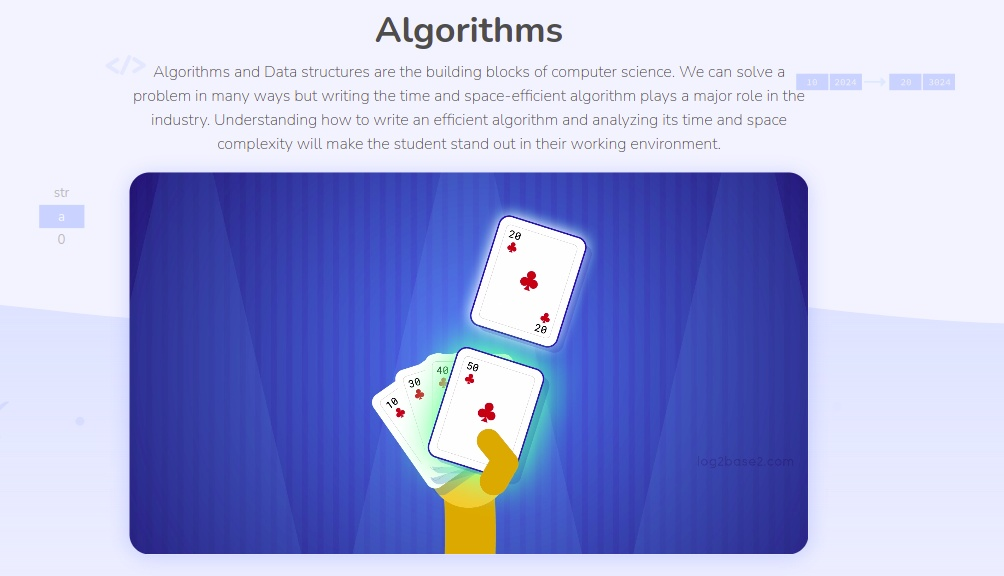138 🔥Harry Potter

Nov 30, 2022

# Algorithms

Algorithms and Data structures are the building blocks of computer science. We can solve a problem in many ways but writing the time and space-efficient algorithm plays a major role in the industry. Understanding how to write an efficient algorithm and analyzing its time and space complexity will make the student stand out in their working environment.

## Course Features

#### Start Learning From Scratch

Our courses are designed in such a way that even an absolute beginner can start to learn and solve problems.

#### Puzzles & Practice Problems

Once you learned the concepts, you can test your knowledge by solving puzzles and practice problems.

We will incrementally add more content to this package. And the new contents will be automatically added to your account.

#### Course Completion Certificate

Once you complete all learning modules and practice sessions. Then you will get the course completion certificate.

#### Trial Videos

• Why should we learn algorithms?
• Bubble sort
• Dynamic Programming
• Divide and Conquer Strategy

#### Introduction to Algorithms

• How to write the algorithms?
• How to analyse the algorithms?
• Time and Space Complexity Analysis - Part 1
• Time and Space Complexity Analysis - Part 2
• Why analysing time complexity is important

#### Searching Algorithms

• Linear Search - Implementation, Time & Space complexity
• Binary Search - Implementation, Time & Space complexity

#### Hashing

• Introduction to hashing and collision
• Collision avoidance using linear probing
• Implement hash table with linear probing - Insert
• Implement hash table with linear probing - Delete
• Implement hash table with linear probing - Search
• Open Hashing or Separate Chaining

#### Sorting

• Introduction to selection sort
• Selection sort
• Selection sort time and space complexity
• Introduction to bubble sort
• Bubble sort
• Bubble sort time and space complexity
• Introduction to insertion sort
• Insertion sort
• Insertion sort time and space complexity
• Introduction to Binary Heap
• Heapify
• Heap Sort
• Quick Sort Introduction
• Implementation of Partion function - Quick Sort
• Merge Sort I
• Merge Sort II
• Implementation of Merge Function

#### Divide and Conquer Strategy

• Introduction to Divide and Conquer Strategy
• Binary Search using Divide and Conquer Strategy

#### Optimization Problem

• What is an optimization problem? How to solve it?

#### Dynamic Programming

• Introduction to Dynamic Programming
• Memoization: Avoid Recomputation Issue in Top-Down Approach
• Implementation of Nth Fibonacci: Bottom-Up approach
• Implementation of Nth Fibonacci: To-Down Approach with Memoization

#### Greedy Algorithms

• Introduction to Greedy Algorithms
• Minimum Coin Exchange Problem
• Greedy can be Wrong? Yes!
• Implementation of Minimum Coin Exchange Problem

#### Graph Traversa

• Depth First Search (DFS)

Zip/rar files password can be one of these :- FreeCourseUniverse / CheapUniverse
Membership### Harry Potter

Hey Guys We are Tech Enthusiasts and we know knowledge is key to success ! We are here to open path to your success by providing what you want. Today education == business. Our moto is education should be accessible by any person who is not able to purchase overpriced content.

### 0 Comment

New Forum

All TakenDown courses are available here

Affiliate